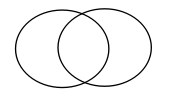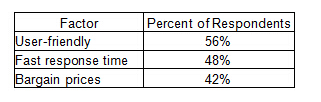考过的GMAT的同学都在说，GMAT数学考试难度与日俱增，GMAT数学这个对中国考生颇具优势的部分，也越来越难拿到高分。为了帮大家更好的搞定GMAT数学，小编今天来为大家总结一下GMAT数学中的集合和函数问题。

一、集合Sets

集合是指具有某种特定性质的具体的或抽象的对象汇总而成的集体。其中，构成集合的这些对象则称为该集合的元素。

并集(union)：给定两个集合A，B，把他们所有的元素合并在一起组成的集合，叫做集合A与集合B的并集，记作A∪B，读作A并B。

交集(intersection)：两个集合和的交集是含有所有既属于又属于的元素，而没有其他元素的集合，记作A∩B，读作A交B。

补集(complementary)：一般地，设S是一个集合，A是S的一个子集，由S中所有不属于A的元素组成的集合，叫做子集A在S中的绝对补集(简称补集或余集)。

集合的计算公式：

A∪B=A+B-A∩B

A∪B∪C=A+B+C-A∩B-B∩C-A∩C+A∩B∩C

韦恩图Venn diagram：例题1：

Is the number of members of Club X greater than the number of members of Club Y ?

(1) Of the members of Club X, 20 percent are also members of Club Y.

(2) Of the members of Club Y, 30 percent are also members of Club X.

A.Statement (1) ALONE is sufficient, but statement (2) alone is not sufficient.

B.Statement (2) ALONE is sufficient, but statement (1) alone is not sufficient.

C.BOTH statements TOGETHER are sufficient, but NEITHER statement ALONE is sufficient.

D.EACH statement ALONE is sufficient.

E.Statements (1) and (2) TOGETHER are NOT sufficient.

【解析】C

条件1：X∩Y=20%X，不充分

条件2：X∩Y=30%Y，不充分

结合：20%X=30%Y，X>Y，充分

例题2：

Last year 26 members of a certain club traveled to England, 26 members traveled to France, and 32 members traveled to Italy. Last year no members of the club traveled to both England and France, 6 members traveled to both England and Italy, and 11 members traveled to both France and Italy. How many members of the club traveled to at least one of these three countries last year?

A52

B67

C71

D73

E79

【解析】B

E=26,F=26,I=32

E∩F=0，E∩I=6，F∩I=11

E∪F∪I=26+26+32-6-11=67

例题3：The table gives three factors to be considered when choosing an Internet service provider and the percent of the 1,200 respondents to a survey who cited that factor as important. If 30 percent of the respondents cited both “user-friendly” and “fast response time,” what is the maximum possible number of respondents who cited “bargain prices,” but neither “user-friendly” nor “fast response time?”

A312

B336

C360

D384

E420

【解析】A

设“user-friendly”为A集合，“bargain prices”为B集合，“fast response time”为C集合

A∩C=30%，A∪C=56%+48%-30%=74%

A∪B∪C≤100%

只属于B集合≤26%→1200*26%=312

例题4：

Club

number of students

Chess

40

Drama

30

Math

25

The table above shows the number of students in three clubs at McAuliffe School. Although no student is in all three clubs, 10 students are in both Chess and Drama, 5 students are in both Chess and Math, and 6 students are in both Drama and Math. How many different students are in the three clubs?

A.68

B.69

C.74

D.79

E.84

【解析】C

题干：C∩D=10,C∩M=5,D∩M=6,C∩D∩M=0

C∪D∪M=C+D+M-C∩D-C∩M-D∩M+C∩D∩M

C∪D∪M=40+30+25-10-5-6=74

二、函数Function

函数的定义：给定一个数集A，假设其中的元素为x。现对A中的元素x施加对应法则f，记作f(x)，得到另一数集B。假设B中的元素为y。则y与x之间的等量关系可以用y=f(x)表示。我们把这个关系式就叫函数关系式，简称函数。函数概念含有三个要素：定义域A、值域C和对应法则f。其中核心是对应法则f，它是函数关系的本质特征。

例题1：

An operation θ is defined by the equation a θ b =(a-b)/(a+b) , for all numbers a and b such that a ≠ –b. If a ≠ –c and a θ c = 0, then c =

A.–a

B.–1/a

C.0

D.1/a

E.a

【解析】E

a θ c=(a-c)/(a+c)=0

a=c

例题2：

If [x] is the greatest integer less than or equal to x, what is the value of [–1.6] + [3.4] + [2.7] ?

A.3

B.4

C.5

D.6

E.7

【解析】A

[–1.6] + [3.4] + [2.7]=-2+3+2=3

例题3：

For any integers x and y, min(x, y) and max(x, y) denote the minimum and the maximum of x and y, respectively. For example, min(5, 2) = 2 and max(5, 2) = 5. For the integer w, what is the value of min(10, w) ?

(1) w = max(20, z) for some integer z.

(2) w = max(10, w)

A. Statement (1) ALONE is sufficient, but statement (2) alone is not sufficient.

B. Statement (2) ALONE is sufficient, but statement (1) alone is not sufficient.

C. BOTH statements TOGETHER are sufficient, but NEITHER statement ALONE is sufficient.

D. EACH statement ALONE is sufficient.

E. Statements (1) and (2) TOGETHER are NOT sufficient.

【解析】D

条件1：如果z>20，w=z>20;若z<20，w=20，所以min(10, w)=10，充分

条件2：w = max(10, w)可知w>10，所以min(10, w)=10，充分

以上就是小编为大家分享的关于GMAT数学中的集合和函数问题的讲解，希望能给大家带来帮助，祝大家能取得一个好成绩

备考推荐：